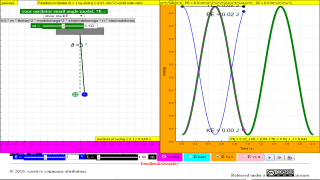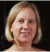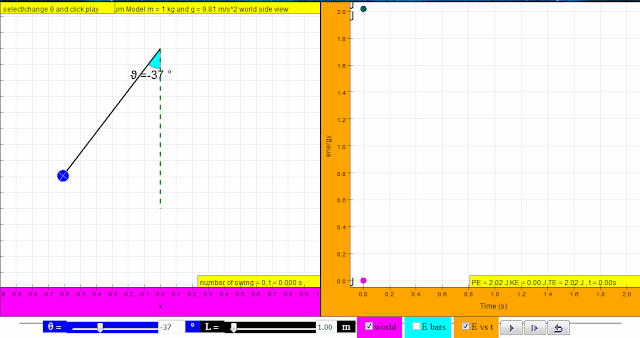## Energy Pendulum Model with Modeling### Translations

Code Language Translator Run
ta ta ta### Software Requirements

SoftwareRequirements

 Android iOS Windows MacOS with best with Chrome Chrome Chrome Chrome support full-screen? Yes. Chrome/Opera No. Firefox/ Samsung Internet Not yet Yes Yes cannot work on some mobile browser that don't understand JavaScript such as..... cannot work on Internet Explorer 9 and below

### CreditsThis email address is being protected from spambots. You need JavaScript enabled to view it.; annecox; tinatan

### end faq

http://iwant2study.org/lookangejss/02_newtonianmechanics_7energyworkpower/ejss_model_SHMxvapendulumjc/SHMxvapendulumjc_Simulation.xhtml

[text]

### For Teachers

INVESTIGATE

Go to the following website to access a simulation about energy of a pendulum: http://tinyurl.com/ast2016-4The left panel shows a pendulum—a mass on a string that can oscillate back and forth.

The right panel allows you to plot one of two types of graphs:

• E bars gives bar graphs that shows the amount of potential and kinetic energy (pe and ke) at any specific moment.
• E vs t graphs the pe and ke of the pendulum over time.

Remember that you can play (►) the simulation by clicking the appropriate button and the pause button ( ▌▌) to pause it.

1. Run several trials with the pendulum, watching the E bars and the E vs t graphs. Note you can have to choose the E bars or the E vs t graphs; you cannot show both at the same time.
1. You can drag the pendulum’s bob to change its position. This changes the initial angle (indicated by the Greek letter θ, theta, and the blue sliding bar at the bottom).
2. You can also change the length (L) of the pendulum using the slider at the bottom of the simulation.
2. Let the pendulum oscillate for a while. Answer the following questions by looking at the E vs t graphs.
3. At what point of the pendulum’s swing is the potential energy the highest?
• Suggested Answer:  at the extreme ends where vertical height of pendulum mass, h is maximum.
4. At what point of the swing is the kinetic energy the highest?
• Suggested Answer: at the middle where the angle of mass with vertical line θ is 0 degree where velocity of mass is maxmum
5. What happens if you change θ (but leave L the same)?
• Suggested Answer: a larger θ will result in a larger maximum potential and kinetic energies.
6. What happens if you change L (but leave initial θ the same)?
• Suggested Answer: a larger L will result in a larger maximum potential and kinetic energies as consequence of a larger change in height due to larger L.

MODEL

The potential energy pe depends on the height above the lowest point h. Set up the applet to show the E bars on the right. Pause the applet at various points. Try to fill up the table below for various values of h. Note that the mass of the bob is 1 kg and that the acceleration of free fall is g = 9.81 m/s2

 Height h in m Weight m g in N Potential energy pe in J 9.81

Time for some more modelling rules!

1. Write down some rules for the pe and ke in the pendulum’s motion. Consider rules in general, and some rules involving θ and L. Here’s an example of a general rule:

“Total energy te is equal to the sum of pe and ke”

The first rule, given above, is a consequence of the principle of conservation of energy. It states that

“energy can be converted from one form to another but cannot be created or destroyed.”

In our oscillating pendulum, energy is converted from potential to kinetic and back again all the time. But energy cannot be destroyed, so the total amount remains fixed.

APPLY

This figure shows the E-t graph for a few swings of a pendulum.1. Can you reproduce this figure? Try adjusting the initial angle and the length of the pendulum to see if you can re-recreate it perfectly.

### Energy transfers and transformations Key inquiry question:

How can we account for the ‘appearance’ and ‘disappearance’ of energy when objects interact with each other?

#### 1. & 2.Work and power

• Work done is defined as the force x distance moved in the direction of the force.

• When we do work, energy is ‘used up’. The amount of work is equal to the energy transferred or used.

• Power is the rate of doing work and is given by: power = work done / time taken (P = W/t).

#### 3. Potential energy and kinetic energy

• Kinetic energy is the energy a body possesses due to its motion.

• Kinetic energy Ek = ½ mv2 .

• Potential energy is the energy stored in a body due to its position, state or shape (e.g. elastic, gravitational, chemical). Gravitational potential energy is the energy which a body possesses due to its position relative to the ground. Gravitational potential energy Ep = mgh.

• The total mechanical energy (Ek and E p) is conserved if no frictional forces are present in a moving system.

#### 4. Energy conversion and conservation

• Energy is defined as the capacity to do work. Energy is transferred when work is done.

• There are different forms of energy e.g. kinetic energy, elastic potential energy, gravitational potential energy, chemical potential energy and thermal energy.

• Energy can be transformed from one form to another but cannot be destroyed or created (principle of conservation of energy).

### Students’ prior knowledge of Energy

#### Primary level:

Students learn that energy:

• is required to make things work or move and energy from most of our energy resources is derived in some ways from the Sun, our primary source of light and heat energy.

• exist in different forms e.g. kinetic energy (movement energy), gravitational potential energy (objects above the ground), elastic potential energy (spring, elastic band), light energy, electrical energy, sound energy, heat energy and chemical energy (as a form of stored energy: food, batteries, fuels).

Students do simple experiments to investigate energy conversion from one form to another e.g. in an electric circuit; learn about sources of energy such as wind, water and fuels, and the need to reduce energy usage in our everyday lives.

#### Lower secondary level:

Students learn that:

• work is the use of a force to move an object.

• work done by a force is defined as force x distance moved in the direction of the force and, based on this definition of work, there are situations involving forces where work is done and where work is not done (calculation only for force parallel to direction of motion) (unit: joule).

• energy is the ability (or capacity) to do work or to produce change (work done = energy used) and there are different forms of energy e.g. kinetic, potential, light and sound.

• energy is conserved and can only change from one form to another (the total amount of energy before and after the change is exactly the same).

• sources of energy include fossil fuels (coal, oil, gas), kinetic energy from water and wind, nuclear, solar, and biomass.

### Students’ common misconceptions and learning difficulties in Energy

Forces between masses, charges and magnets:

Students often think of energy either as a physical substance that flows out of one thing to another or as a kind of force. Work done on a body: Students have difficulty understanding that the work done on a body represents the energy transferred during the interaction between the body and another system, and does not represent energy stored in a body.

Energy is conserved:

Students often think that energy is used up or lost (disappears) during interactions

[text]

### Video

Energy Pendulum Model - Secondary & JC by Dave Lommen

[text]

### Project related:

Understanding Teacher Learning Community as Support for Implementation of Open Source Physics for Conceptual Instruction
Project Number: OER 10/15 GWF
Project Duration: 01 July 2015 - 30 April 2017

### end faq

Rating 5.00 (4 Votes)